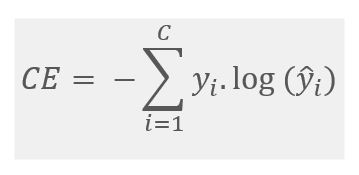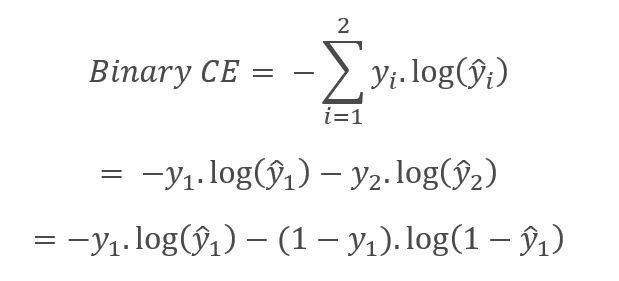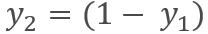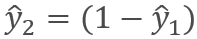Arindam Banerjee — Published On September 28, 2022

This article was published as a part of the Data Science Blogathon.

## Introduction

Have you ever encountered a situation where you felt to use a custom loss function in your machine learning model? Maybe, you had to experiment with a new loss function while writing a research paper or to handle a new business case. Writing a custom loss function for the machine learning models is not very difficult. This article will teach us how to write a custom loss function in Tensorflow. We will write the custom code to implement the categorical cross-entropy loss. Then we will compare its result with the inbuilt categorical cross-entropy loss of the Tensorflow library.

Through machine learning, we try to mimic the human learning process in a machine. Like us, machines also learn from past mistakes. A loss function is used to evaluate the machine’s learning quality. It shows how well the machine learning model can predict the outcome from a given feature set. Building any machine learning model intends to predict the probabilistic target value as accurately as possible. So, the loss function can measure how far the predicted value is from the actual value. The loss function is not fixed and changes based on the task. The goal of an optimization procedure is to minimize the loss function.

## Loss Functions in Tensorflow

Tensorflow is a widely used Python-based machine learning platform. Tensorflow library can be used for developing machine learning models across tasks. But this library has a certain focus on developing deep learning models efficiently. Tensorflow provides many inbuilt and optimized loss functions for developing machine learning models. Some commonly used loss functions in regression tasks are Mean Squared Error (MSE), Mean Absolute Error (MAE), Mean Absolute Percentage Error (MAPE), Huber Loss, etc. Common loss functions in classification tasks are binary cross-entropy, categorical cross-entropy, etc.

## Categorical Cross-entropy

We use categorical cross-entropy loss when we need to predict two or more target classes (multi-class classification). Here the model is trained to predict a class from many classes. If we consider the actual target value is y, the predicted value is, and there are C number of classes, then the Categorical Cross-entropy (CE) loss can be defined as:Usually, an activation function (Sigmoid/Softmax) is applied to the scores before the Cross-entropy Loss is computed. Categorical Cross-entropy loss is a generalized version of Binary Cross-entropy loss. In Binary Cross-entropy loss, C = 2 since there are only two classes. Hence the loss becomes:Since there are only two possible classes,and.

## Custom Loss Function in Tensorflow

We will write the categorical cross-entropy loss function using our custom code in Tensorflow with the Keras API. Then we will compare the result between this custom categorical cross-entropy function and Tensorflow’s inbuilt categorical cross-entropy function.

Once trained, every model produces a predicted value of the target variable. For a loss function, we need the model’s actual value and the predicted value to compare and calculate the loss value. In Tensorflow, we will write a custom loss function that will take the actual value and the predicted value as input. This custom loss function will subclass the base class “loss” of Keras. For best performance, we need to write the vectorized implementation of the function. We will also use basic Tensorflow functions to get benefitted from Tensorflow’s graph feature.

```class Custom_CE_Loss(tf.keras.losses.Loss):
def __init__(self):
super().__init__()
def call(self, y_true, y_pred):
log_y_pred = tf.math.log(y_pred)
elements = -tf.math.multiply_no_nan(x=log_y_pred, y=y_true)
return tf.reduce_mean(tf.reduce_sum(elements,axis=1))```

Here, we can see that the Custom_CE_Loss function is subclassed from the base class “Loss”. We are overriding the call method that takes the true value and predicted value as input. We are also using Tensorflow’s inbuilt math functions for calculating log, multiplication, sum, mean, etc.

The multiply_no_nan function computes the product of x and y and returns 0 if y is zero, even if x is NaN or infinite. The reduce_sum function computes the sum of elements across dimensions of a tensor. The reduce_mean function reduces input_tensor by computing the mean of elements across the dimensions given in the axis.

## Comparing the Custom Loss Function

Let’s assume in a multiclass classification problem, there are five class labels. There are two data points where the target classes are 4 and 1. We need to first convert this class vector (integers) to a binary class matrix using the to_categorical function. The predicted values are in a vector called y_pred, which is the softmax output of a classifier.

```y_true = tf.constant(tf.keras.utils.to_categorical([4, 1]))
y_pred = tf.constant([[0 , .7 , 0 ,0 ,  .3], [0 , .6 , .3 ,0 ,  .1]])```

We will run the Custom_CE_Loss function mentioned above and TensorFlow’s CategoricalCrossentropy loss function and compare the results.

```print('Tensorflow CE : ',tf.keras.losses.CategoricalCrossentropy()(y_true, y_pred).numpy())
print('My CE : ', Custom_CE_Loss ()(y_true, y_pred).numpy())```

Both the results should come as 0.8573992.

Let’s implement this custom loss function in a Neural Network for a multiclass image classification problem. We will use Tensorflow’s pre-trained VGG16 model to classify CIFAR-10 images. The CIFAR-10 dataset consists of 60000 32×32 colour images in 10 classes, with 6000 images per class. There are 50000 training images and 10000 test images.

```import tensorflow as tf
from tensorflow.keras import datasets
from keras.layers import Dense, Dropout, Flatten
from keras.models import Model
​
(train_images, train_labels), (test_images, test_labels) = datasets.cifar10.load_data()
#Normalize the pixel values
train_images, test_images = train_images / 255.0, test_images / 255.0```

The target classes are numerical values of the class label. We need to encode one-hot and make them float.

```train_labels = tf.keras.utils.to_categorical(train_labels)
test_labels = tf.keras.utils.to_categorical(test_labels)
train_labels = tf.convert_to_tensor(train_labels, dtype=tf.float32)
test_labels = tf.convert_to_tensor(test_labels, dtype=tf.float32)```

Let’s load Tensorflow’s pre-trained VGG16 model. We will not include the top layers (full-connected layers) since we will train them for this task (CIFAR-10 classification). The convolution and pooling layers are all kept pre-trained and this is called transfer learning.

```base_model = tf.keras.applications.vgg16.VGG16(input_shape = (32, 32, 3),
include_top = False,
weights = 'imagenet')
base_model.trainable=False
model=base_model.output
model=Flatten()(model)
model=Dense(4096, activation='relu')(model)
model=Dropout(rate=0.5)(model)
model=Dense(4096, activation='relu')(model)
model=Dropout(rate=0.5)(model)
model=Dense(10, activation='softmax')(model)
model=Model(inputs=base_model.inputs, outputs=model)
model.summary()```

Let’s compile the model with this custom cross-entropy loss taking “accuracy” as metric. We will run the training for 10 epochs and evaluate the model on the test dataset.

```model.compile(optimizer='adam',
loss=Custom_CE_Loss(),
metrics=['accuracy'])
history = model.fit(train_images, train_labels, epochs=10,
validation_data=(test_images, test_labels))
test_loss, test_acc = model.evaluate(test_images,  test_labels, verbose=2)```

## Conclusion

This article taught us about loss functions in general, common loss functions, and how to define a loss function using Tensorflow’s Keras API. We wrote custom code for the categorical cross-entropy loss and then compared the result with the same loss function available in Tensorflow. We also used this custom-written categorical cross-entropy loss in training a Neural Network model. Key takeaways from this article are:

• We learned what the categorical cross-entropy loss is, how it works and how it generalizes the binary cross-entropy loss.
• We learned to write a categorical cross-entropy loss function in Tensorflow using Keras’s base Loss function.
• We compared the result with Tensorflow’s inbuilt cross-entropy loss function.
• We implemented the custom loss function for a multiclass image classification problem using a pre-trained VGG16 model.

Like the cross-entropy loss recreated above, any other loss function can also be written easily in Tensorflow.

Thanks for reading! Please let me know in the comment section if you have any questions.

References

1. Tensorflow Loss functions: https://www.tensorflow.org/api_docs/python/tf/keras/losses
2. Tensorflow Categorical Cross-Entropy loss: https://www.tensorflow.org/api_docs/python/tf/keras/losses/CategoricalCrossentropy